# Trigonometric Quiz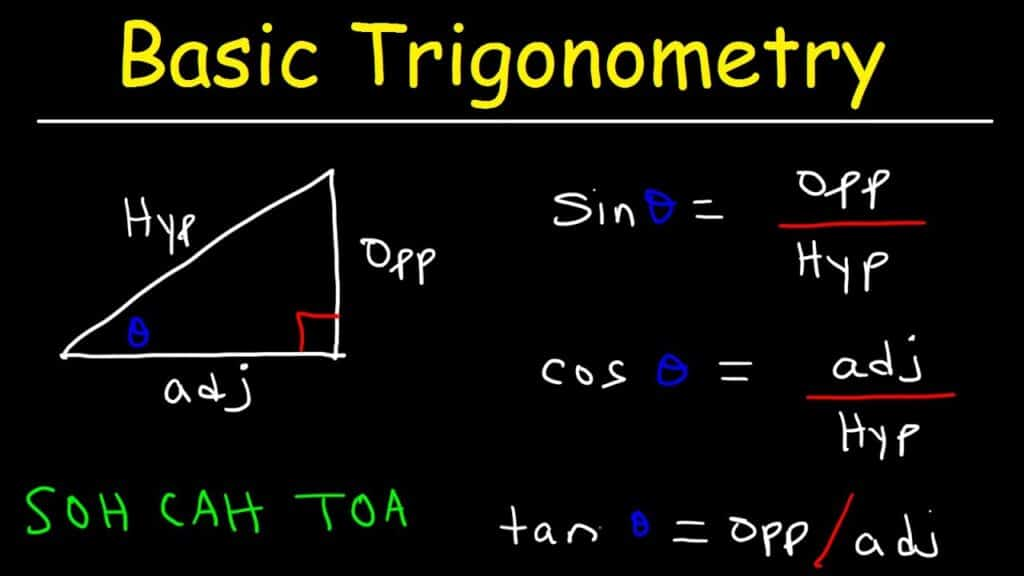We thoroughly check each answer to a question to provide you with the most correct answers. Found a mistake? Let us know about it through the REPORT button at the bottom of the page.

Trigonometric Quiz1 How do you find the inverse of sin in your calculator?

• sin-1
• alpha, sin-1
• 2nd key, sin1
• 2nd key, sin-1

2. How do you find the inverse of tangent in your calculator?

• 2nd key, tan1
• tan-1
• 2nd key, tan-1

3. How do you find the inverse of cosine in your calculator?

• 2nd key, cos1
• cos-1
• alpha, cos-1
• 2nd key, cos-1

4. Value of , for = 1 Where 0° < < 90° is:

• 30
• 60
• 45
• 135

5. Value of sec2 26° – cot2 64° is:

• 0
• 1
• -1
• 2

6. Product tan 1° tan 2° tan 3°………….. tan 89° is:

• 1
• 0
• -1
• 90

7. Find t to the nearest degree using sin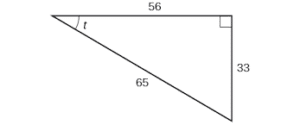• 40
• 41
• 30
• 31

8. Find a to the nearest degree using cosin a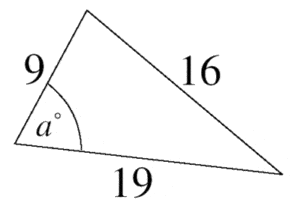• 55
• 56
• 58
• 65

9. If sin (A + B) = 1 = cos (A – B) then

• A=B=90
• A=B=45
• A=B=0

10. What is the inverse cosine?

• cos-1
• cos-2
• cos1

11. The value of 5 cos 0^0 + sin 90^0 is:

• 5
• 6
• 7
• 8

12. What is the inverse sin?

• cos-1
• sin-1
• sin1

13. How does the value of cos x varies when x increases from 0^0 to 90^0:

• Decreases
• Increases
• Increases and then decreases

14. What is the inverse tangent?

• tan1
• sin-1
• tan-1

15. Find the length of H. Round to the nearest tenth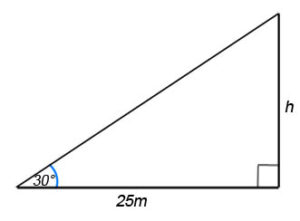• 14.6
• 15.2
• 14.4
• 14.8

16. Find the value of sin^2 54^0 – cos^2 36^0

• -1
• 1
• 0

17. Find Y. Round to the nearest tenth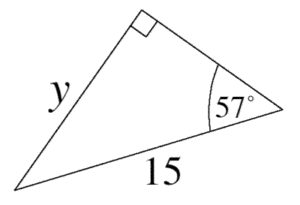• 15.9
• 13.2
• 7.4
• 12.5

18. Cos 45^0 + tan 45^0 – sin 45^0 is:

• 1
• -1
• 2
• -2

19. Find x. Round to the nearest tenth.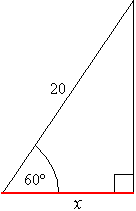• 14.6
• 13.3
• 10.0

20. Sin =?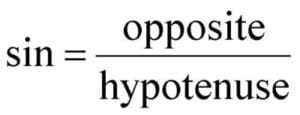• Hypotenuse / Opposite
• Opposite / Hypotenuse

21. Tangent = ?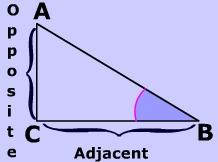22. Find K. Round to the nearest tenth.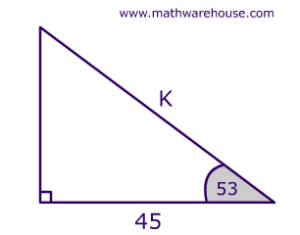• 45.9
• 43.8
• 74.8